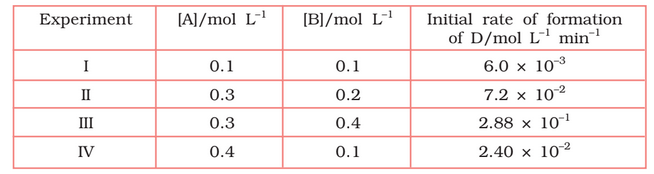# 4.11   The following results have been obtained during the kinetic studies of the reaction:              2A + B  C + DDetermine the rate law and the rate constant for the reaction.

Let assume the rate of reaction wrt A is  and wrt B is  . So, the rate of reaction is expressed as-
Rate =

According to given data,
these are the equation 1, 2, 3 and 4 respectively

Now,  divide the equation(iv) by (i) we get,

from here we calculate that

Again, divide equation (iii) by (ii)

from here we can calculate the value of y is 2

Thus, the rate law is now,
So,

Hence the rate constant of the reaction is

Exams
Articles
Questions